# Logistic regression

## Summary

Item Value
Type of variable predicted Binary (yes/no)
Format of prediction Probabilistic. Rather than simply returning a binary answer, the prediction gives the respective probabilities of the two answers.
Functional form of model Computes the probability by applying the logistic function to a linear combination of the features. The coefficients used in the linear combination are the unknown parameters that need to be determined by the learning algorithm. It is an example of a generalized linear model.
The parameters are sometimes called the model weights, with each model weight associated to a particular feature for which it is the coefficient. The feature for which the model weight is zero can be thought of as not being part of the model, since its value plays no role in the prediction. We sometimes say that the features with nonzero model weights are features "picked" by the training.
Typical cost function As with most probabilistic binary prediction models, logistic regression models are typically scored using the logarithmic cost function. However, they could in principle be scored using the squared error cost function. Note that this still wouldn't be least-squares regression, because the least-squares is being computed after applying the logistic function.
Typical regularization choices Both$L^1$- and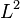$L^2$-regularization, as well as combined regularization using$L^1$ and$L^2$ terms, are common.
Learning algorithms See here for more (to eventually fill in here).

## Definition

The term logistic regression is used for a model as well as the act of finding the parameters of the model whose goal is to predict binary outputs. It is therefore better viewed as solving a classification problem than a regression problem. However, because the model shares many basic components with linear regression, and is an example of a generalized linear model, it has historically gone by the name of logistic regression.

The logistic regression problem attempts to predict a binary output (yes/no) based on a set of inputs (called features). Rather than just predicting a yes/no answer, the logistic regression problem predicts a probability of yes. This is a number in$[0,1]$. By using a threshold probability (such as 0.5, or another value depending on what sorts of risks we want to avoid) this can make a yes/no prediction.

The probability is computed as follows:

Probability = logistic function evaluated at (linear combination of features with initially unknown parameters)

The logistic function is the function:$g(x) = \frac{1}{1 + e^{-x}}$

The values of the unknown parameters are determined empirically so as to best fit the training set.

### Cost function used

The typical cost function used is the logarithmic cost function (also known as logarithmic scoring): This assigns a score of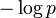$-\log p$ if the event happened and a score of$-\log (1 - p)$ if the event did not happen. The lower the score, the better. The logarithmic scoring rule is proper: if the true probability is$q$, then the score is minimized by predicting$p = q$.

Note that if we could predict whether or not the event will happen with perfect confidence, the logarithmic score would evaluate to 0.

The logarithmic cost function is computed for each of the predictions made by the logistic regression model. We then average the values of the cost functions across all instances to obtain the logarithmic cost function for the specific choice of parameter values on the specific data set.

There are two standard choices of labels for describing whether the event did or did not occur. One choice is to assign a label of 0 if the event did not occur and 1 if the event occurred. Another choice is to assign a label of -1 if the event did not occur and 1 if the event occurred.

#### Closed form expression for cost function using 0,1-encoding

Suppose we assign a label$y$ with value 0 if the event did not occur and 1 if the event occurred. Then, if$p$ is the predicted probability, the score associated with$p$ is: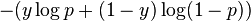$-(y \log p + (1 - y)\log(1 - p))$

Suppose there are$m$ data points. The probability vector is the vector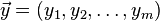$\vec{y} = (y_1,y_2,\dots,y_m)$ and the probability vector is the vector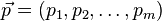$\vec{p} = (p_1,p_2,\dots,p_m)$. The cost function is:$\frac{1}{m} \left[\sum_{i=1}^m -(y_i \log p_i + (1 - y_i)\log(1 - p_i))\right]$

#### Closed form expression for cost function using -1,1-encoding

Suppose we assign a label$l$ with value -1 if the event did not occur and 1 if the event occurred. Then, if$p$ is the predicted probability, the score associated with$p$ is: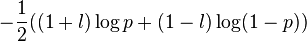$-\frac{1}{2} ((1 + l) \log p + (1 - l)\log(1 - p))$

### Description as a generalized linear model

The logistic regression model can be viewed as a special case of the generalized linear model, namely a case where the link function is the logistic function and where the cost function is the logarithmic cost function.

The inverse of the logistic function is the log-odds function, and applying it to the probability gives the log-odds (logarithm of odds). Explicitly, we have: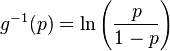$g^{-1}(p) = \ln \left( \frac{p}{1 - p}\right)$

Therefore, the logistic regression problem can be viewed as a linear regression problem:

Log-odds function = Linear combination of features with unknown parameters

However, the cost function now changes as well: we now need to apply the logistic function and then do logarithmic scoring to compute the cost function.

## Computational format

The computational format for a logistic regression is as follows. Note that there may be variations in terms of the roles of rows and columns. We follow the convention of using column vectors and having the matrix multiplied on the left of the vector.

Some notation:

•$m$ denotes the number of examples (data points).
•$n$ denotes the number of features, or equivalently, the number of parameters. Note that the number of elementary features need not equal$n$. The "features" we are referring to are expressions in the elementary features that we can use as the spanning set for our arbitrary linear combinations whose coefficients are the unknown parameters we need to find.
•$X$ is the data matrix or design matrix of the regression.$X$ is a$m \times n$ matrix. Each row of$X$ corresponds to one example. Each column of$X$ corresponds to one feature (not necessarily an elementary feature) and hence also to one coordinate of the parameter vector (the coefficient on that feature). The entry in a given row and given column is the feature value for that example.
• The vector of labels (or actual outputs) is a$m$-dimensional vector. If we use the 0-1 convention, this is a vector all of whose coordinates are either 0 or 1. If we use the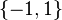$\{ -1,1 \}$-convention, this is a vector all of whose coordinates are either -1 or 1. For convenience on this page, we'll denote the former vector by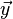$\vec{y}$ and the latter by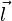$\vec{l}$. We have the relations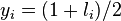$y_i = (1 + l_i)/2$, and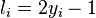$l_i = 2y_i - 1$, for all$i$.
• The parameter vector is a$n$-dimensional vector. We will denote it as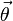$\vec{\theta}$.

The predicted probability vector is given as: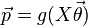$\vec{p} = g(X \vec{\theta})$

where$g$ is the logistic function and is applied coordinate-wise.

## Examples of feature sets and models

### Empty feature set and empty model

The "empty model" for a logistic regression problem is the model with no features, or alternatively, the model where all the features have zero model weights. The linear combination generated for any example is zero, so the probability predicted for any example is the logistic function applied at 0, which is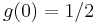$g(0) = 1/2$.

The logarithmic cost function for each example is therefore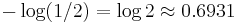$-\log(1/2) = \log 2 \approx 0.6931$, and hence, so is the arithmetic mean. This is treated as a baseline for the logarithmic loss on logistic regression models and for any binary classification models predicting a probability; any logistic regression model that is trained properly should provide a lower (better) cost than the empty model.

Standard choices of regularization such as$L^1$ (lasso),$L^2$ (ridge regression), and elastic net (a mix of the two) all give their lowest penalty of zero to the empty model. Therefore, in general, if the event occurs about half the time and none of the features being trained on have any signal useful for predicting the outcome, a regularized logistic regression will converge to the empty model (however, note the caveat on the bias term below).

### Single-feature bias or intercept model

This is a model with a single nonzero model weight, corresponding to a feature that is 1 on all examples. If this weight is$w$, then the linear combination is$w$ on each example, and the predicted probability works out to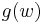$g(w)$.

If this model is trained without regularization on a training set, then the weight$w$ that it learns is$g^{-1}(q)$, where$q$ is the fraction of positive examples in the training set. If the training set is sufficiently large and representative, then$q$ equals the probability of occurrence of the event, so the model essentially predicts the probability of occurrence (the base rate) without trying to figure out which examples are more or less likely to be positive.

In the case of regularization such as$L^1$,$L^2$, or elastic net, the weight$w$ learned is between$0$ and$g^{-1}(q)$, where its exact position is determined by the strength of the regularization term (after normalizing by the number of examples); the larger the regularization terms, the closer$w$ is to zero. In particular, the greater the number of examples, the closer we get to$g^{-1}(q)$, holding the regularization term constant. This makes sense from the perspective of regularization as Bayesian prior: with small amounts of data we gravitate toward the Bayesian prior of even odds, whereas with a large amount of data we gravitate toward the frequency seen in the data.

Specifically, for the case of elastic net regularization with$L^1$-parameter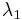$\lambda_1$ and$L^2$-parameter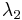$\lambda_2$, and a total of$m$ examples, we have to pick$w$ that minimizes:$-q \log(g(w)) - (1 - q) \log(1 - g(w)) + \frac{\lambda_1}{m} |w| + \frac{\lambda_2}{m} w^2$

Taking derivatives and finding critical points, we get:$g(w) - q + \frac{\lambda_1}{m} \operatorname{sgn}(w) + \frac{2\lambda_2}{m} w = 0$

There is no analytical solution to this but it can be solved using numerical techniques.

### Bias feature and a single binary feature

Consider a logistic regression model with a bias feature and a single binary feature (that can be either 0 or 1). Assume we have enough training data, and the binary feature is both zero and nonzero on enough examples.

There are two ways of operationalizing this. One is to train it as a two-feature model, with one bias feature and the single binary feature. Another is to train it as a three-feature model, with one bias feature, the binary feature, and its complement. The latter approach has a linear relation between features (the binary feature and its complement add up to 1). However, it allows for a more clean-to-interpret model.

Assuming no regularization:

• With the two-feature model, the model weight learned on the bias feature is the log-odds of the probability of the event occurring if the single binary feature is off, and the model weight learned on the binary feature is the correction to the log-odds caused by the binary feature being true.
• With the three-feature model, the model weight learned on the bias feature is the log-odds of the overall probability, independent of whether the binary feature is true or false. The other two model weights give the respective corrections to the log-odds probability from the feature being true and false. These two model weights are of opposite sign, and they can be deduced from one another (but they are not literally negatives of each other, because (a) the feature may not be true and false the same amount of time, so there is a skew, and (b) these are additive corrections on log-odds not on probability itself, so the linearity is not preserved). In particular, the magnitude of the weight should generally be higher for the rarer of the two cases(since this gives more unique information, and is therefore expected to cause a larger update) but that is not always true.

In particular, if knowledge of the binary feature does not change our probability estimate, then the weight learned on the feature and/or its complement is zero.

## Unique property of the logistic link function

The logistic function is not the only possible choice of link function that can be used to apply generalized linear models to probabilistic binary classification; another choice is the normal CDF, used in probit regression.

However, the logistic link function is the only link function$g$ that satisfies the property of taking the value 1/2 at 0 (which is necessary for the symmetric sigmoidal shape we seek) and satisfies the condition that, if the true probability is$q$ and the linear combination in question is$w$, then the derivative of the logarithmic cost function is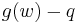$g(w) - q$, and therefore the second derivative is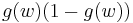$g(w)(1 - g(w))$ which is positive and bounded by$1/4$. This is because of the differential equation it satisfies. This provides an easy proof of convexity as well as bounded second derivative, and shows that gradient descent can be applied.

## Relation with other forms of machine learning

### Probit regression

Aspect How they're similar How they're different
Generalized linear models used for probability prediction for binary classification Both fit the description; the link function for logistic regression is the logistic function and the link function for probit regression is the normal CDF. The logistic link function is the unique function where the cost grows quadratically with distance from the true probability, or equivalently, the marginal cost is linear in distance from the true probability.
Use of gradient descent Logistic regression is a convex optimization problem with a globally bounded second derivative, therefore gradient descent works. What about probit?  ??

### Linear regression

Logistic regression and linear regression are related in the following ways:

Aspect How they're similar How they're different
Generalized linear models, so linear dependence on inputs Both are examples of generalized linear models For linear regression, the link function is the identity function and the typical choice of cost function is the squared error cost function. In the case of logistic regression, the link function is the logistic function and the typical choice of cost function is the logarithmic cost function.
Prediction of continuous variables Prima facie, both of them output variables that take continuous values Linear regression outputs a continuous variable that is the estimate of the output being predicted.
The continuous variable output by logistic regression is the probability associated with a binary classification problem.

### Support vector machines

Logistic regression and the support vector machine (SVM) regression method are related in the following ways:

Aspect How they're similar How they're different
Binary classification Both logistic regression and support vector model are approaches to tackling binary classification. Logistic regression outputs a probability, whereas support vector models output a yes/no answer. Support vector machines can be construed as giving an output describing the confidence of a classification, but this is not explicitly translated into a probability. Note that the linear SVM result can be interpreted as a result for the logistic regression problem, and running linear SVM and logistic regression on the same data set can yield very similar results.

### Artificial neural networks

Artificial neural networks are a more complicated type of machine learning setup that is capable of learning more complex functions. The individual units in an artificial neural network, called artificial neurons, can in principle be chosen to be any functions, but the typical choice is to choose each of them as a logistic regression model. In other words, the output of each artificial neuron is obtained by computing the logistic function of a linear combination (via an unknown parameter vector) of the inputs.

### Maximum entropy (MaxEnt) models

Maximum entropy models generalize logistic regression to particular types of classification problems where the relative probabilities of the discrete classes satisfy a particular kind of mathematical relationship (the need for a constraint on the relationship arises only when there are three or more different possibilities; no assumptions are necessary in the binary case).